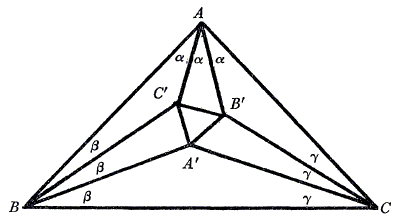## Morley's MiracleR. J. Webster's Proof

### Theorem

 The three points of intersection of the adjacent trisectors of the angles of any triangle form an equilateral triangle.

### Proof

This proof appeared in Mathematics Magazine, Vol. 43, No. 4 (Sep., 1970), pp. 209-210.

Let ABC be a triangle with inradius r and circumradius R, and let the adjacent trisectors of angles A, B, C meet in A', B', C' as illustrated below. Morley's theorem states that ΔA'B'C' is equilateral. The proofs of this theorem, which are usually given, do not include calculation of the side of this triangle.In this note we prove Morley's theorem by showing that the side of this triangle is 8R sin(A/3)sin(B/3)sin(C/3). This is a particularly interesting result, when one recalls the formula r = 4R sin(A/2)sin(B/2)sin(C/2).

In any triangle ABC, we have a = 2R sin(A), etc. Let A = 3α, B = 3β, C = 3γ. Then applying the sine rule to A'BC we obtain

 A'B = 2R sin(3α) sinγ / sin(β + γ) = 2R (4sinα sin(60° + α) sin(60° - α) sinγ) / sin(60° - α) = 8R sinα sinγ sin(60° + α).Similarly, BC' = 8R sinα sinγ sin(60° + γ). Consider now triangle DEF shown above, where DE = 8R sinα sinγ sin(60° + α). It follows, using the sine rule on DEF, that EF = 8R sinα sinγ sin(60° + γ) and DF = 8R sinα sinβ sinγ. However, triangles A'BC' and DEF are congruent by SAS, so A'C' = DF = 8R sinα sinβ sinγ Thus, by the symmetry of the expression, the triangle A'B'C' is equilateral and Morley's theorem is proved.Editorial Note: Professor C. N. Mills of Illinois State University at Normal as a tour de force found above expression for a side of the Morley triangle by a straightforward use of elementary Cartesian analysis! His complete proof required some twenty 8½×11 sheets of paper.### Morley's Miracle

#### Invalid proofs